# Solving Linear Equations With The Distributive Law

in Mathematics10 days ago

Hello everyone. In this math post, I cover solving linear equations with the distributive law. This is suitable for early high school mathematics.Pixabay Image Source

## Topics

• Review Of Solving Linear Equations
• Review Of Distributive Law
• Solving Linear Equations With The Distributive Law
• Practice Problems
• Solutions To Practice Problems

## Review Of Solving Linear Equations

When it comes to solving linear equations we seek to find the value of the unknown (such as `x`) which makes the equation hold true. For the equation `x - 7 = 8`, the value of the unknown `x` is 15.

Consider a more involved example where we have the equation `2x - 7 = 15`. To find the value of `x` here. We add 7 to both sides to "remove" the negative seven from the left side. This leaves us with: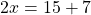Computing the right side of 15 and 7 gives 22. We now have `2x = 22`. To find the value of just `x` itself, divide by 2 on both sides. The value of the unknown `x` is just 11.

## Review Of The Distributive Law

The distributive law from algebra allows for multiplication of a single term monomial with a polynomial. One example would be `5(x + 3)`. Multiplying the 5 through each term in (x + 3) with the distributive law yields `5x + 15`.Duckduckgo Image Source

The above example dealt with a monomial with a binomial. What if the binomial was something else that was longer. Let's take a look at another example.

Using the distributive law on `8(x + 2y + z)` gives `8x + 16y + 8z`. The distributive law idea still holds. It is just there are more computations involved.

## Solving Linear Equations With The Distributive Law

There are times when you need to certain linear equations that require the distributive law. Once you have a good grasp on using the distributive law you can easily solve for unknowns. Let's look at a few examples.

Example One

Solve for x in the equation `9(x + 2) = 22`.

As soon as you see something like a number multiplied by a binomial in brackets, think of the distributive law first. (You could divide by 9 both sides and subtract by 2 to solve as an alternate approach.)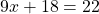Isolate for 9x by subtracting 18 on both sides.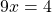Since we want to solve for a single `x` divide both sides of the equation by 9. The answer for `x` is four ninths.

Example Two

This second example is a bit more involved. Solve for `x` in the equation 3(x - 7) = 5 + 8(x + 3).

Use distributive law on both sides accordingly. The equation then becomes: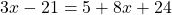Add the 2 and 24 from the right side.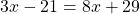Collect the `x` terms in one side and the numbers to the other side.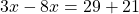Simplify the like terms.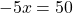To solve for `x`, divide both sides by negative 5.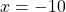## Practice Problems

In each question solve for the value of `x`.

1. 3(x - 2) = 12

2. -5(x - 1) = 2

3. 10x - 2 = 3(x - 4)

4. 7(x - 2) = 9(2x + 1)

5. 2(x + y) = 3(x - y)

## Solutions To Practice Problems

1. 6

2. 3/5

3. -10/7

4. -23/11

5. 5yPixabay Image Source